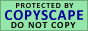OPERATORS
An operator is a symbol specifying an action that is performed on one or more expressions. SQL Server operators can categories into Arithmetic Operators, Assignment Operator, String Concatenation Operator, Comparison Operators, Bitwise Operators, Unary Operators, Compound Operators, Scope Resolution Operator, Logical Operators and SET Operators.

Arithmetic Operators

Arithmetic operators are used to execute mathematical operations on two expressions of one or more of the data types of the numeric data type category.

 Symbol Name Description + Add To Add two or more Numeric and Date value. - Subtract To Subtract two or more Numeric and Date value. * Multiply To Multiply two or more Numeric value. / Divide To Divide one to another Numeric value. % Modulo To find out remainder value when divide one to another Numeric value.

Assignment Operator

Assignment operator is used to stores a constant or variant value in the specified variable.

 Symbol Name Description = Equal To stores a constant or variant value in the specified variable.

String Concatenation Operator

String concatenation operator is used combine two or more string values into one string value.

 Symbol Name Description + Plus Sign To combine two or more string values into one string value.

Comparison Operators

Comparison operators are used to checks a condition between two variables or expressions values that returns TRUE or FALSE Boolean data. Comparison operators cannot checks with text, ntext or image data types.

 Symbol Name Description = Equals To returns TRUE when left side variable or expression is equal with right side variable or expression value. > Greater Than To returns TRUE when left side variable or expression is greater than with right side variable or expression value. < Less Than To returns TRUE when left side variable or expression is less than with right side variable or expression value. >= Greater Than or Equal To To returns TRUE when left side variable or expression is greater than or equal with right side variable or expression value. <= Less Than or Equal To To returns TRUE when left side variable or expression is less than or equal with right side variable or expression value. <> Not Equal To To returns TRUE when left side variable or expression is not equal with right side variable or expression value. != Not Equal To To returns TRUE when left side variable or expression is not equal with right side variable or expression value. !< Not Less Than To returns TRUE when left side variable or expression is not less than with right side variable or expression value. !> Not Greater Than To returns TRUE when left side variable or expression is not greater than with right side variable or expression value.
Next >>Your Comment To AUTHORDownload e-Book